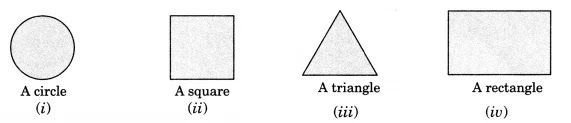NCERT Solutions for Class 7 Maths Chapter 15 Visualising Solid Shapes Ex 15.4

# NCERT Solutions for Class 7 Maths Chapter 15 Visualising Solid Shapes Ex 15.4

## NCERT Solutions for Class 7 Maths Chapter 15 Visualising Solid Shapes Ex 15.4

NCERT Solutions for Class 7 Maths Chapter 15 Visualising Solid Shapes Ex 15.4 are the part of NCERT Solutions for Class 7 Maths. Here you can find the NCERT Solutions for Class 7 Maths Chapter 15 Visualising Solid Shapes Ex 15.4.

### Ex 15.4 Class 7 Maths Question 1.

A bulb is kept burning just right above the following solids. Name the shape of the shadows obtained in each case. Attempt to give a rough sketch of the shadow. (You may try to experiment first and then answer these questions).

Solution:
When the light bulb is kept just above the given solids, we get the following shapes as shadows.
(i) A ball: The shape of the shadow obtained is a circle.
(ii) A cylindrical pipe: The shape of the shadow obtained is a rectangle.
(iii) A book: The shape of the shadow obtained is a rectangle.

### Ex 15.4 Class 7 Maths Question 2.

Here are the shadows of some 3-D objects, when seen under the lamp of an overhead projector. Identify the solid(s) that match each shadow. (There may be multiple answers for these!)Solution:
(i) The given shadow corresponds to a sphere, a cylinder or a cone.
(ii) The given shadow corresponds to a cube.
(iii) The given shadow corresponds to a prism or a pyramid.
(iv) The given shadow corresponds to a cuboid or a cylinder.

### Ex 15.4 Class 7 Maths Question 3.

Examine if the following are true statements:
(i) The cube can cast a shadow in the shape of a rectangle.
(ii) The cube can cast a shadow in the shape of a hexagon.

Solution:
(i) The given statement is true.
(ii) The given statement is false.

You can also like these:

NCERT Solutions for Maths Class 7

NCERT Solutions for Maths Class 8

NCERT Solutions for Maths Class 9

NCERT Solutions for Maths Class 10

NCERT Solutions for Maths Class 11

NCERT Solutions for Maths Class 12